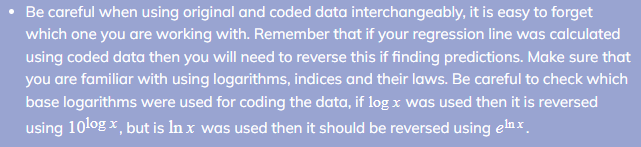# AQA A Level Maths: Statistics复习笔记2.5.1 PMCC & Non-linear Regression

### Product Moment Correlation Coefficient (PMCC)

#### What is the product moment correlation coefficient?

• The product moment correlation coefficient (PMCC) is a way of giving a numerical value to linear correlation of bivariate data
•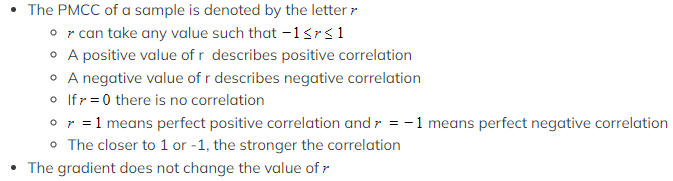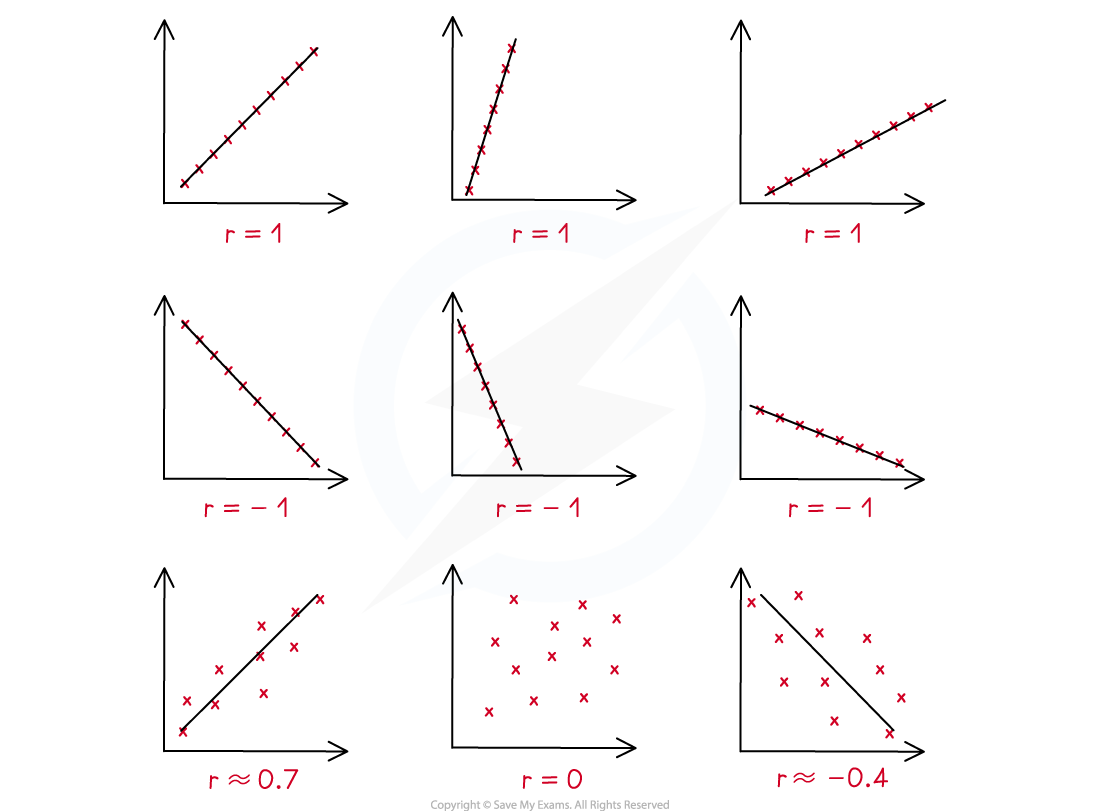#### Worked Example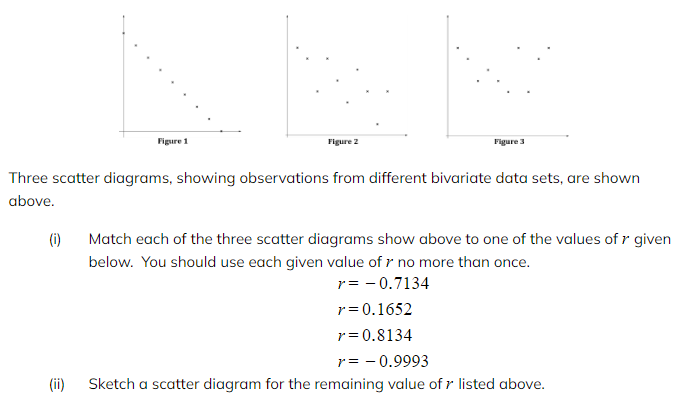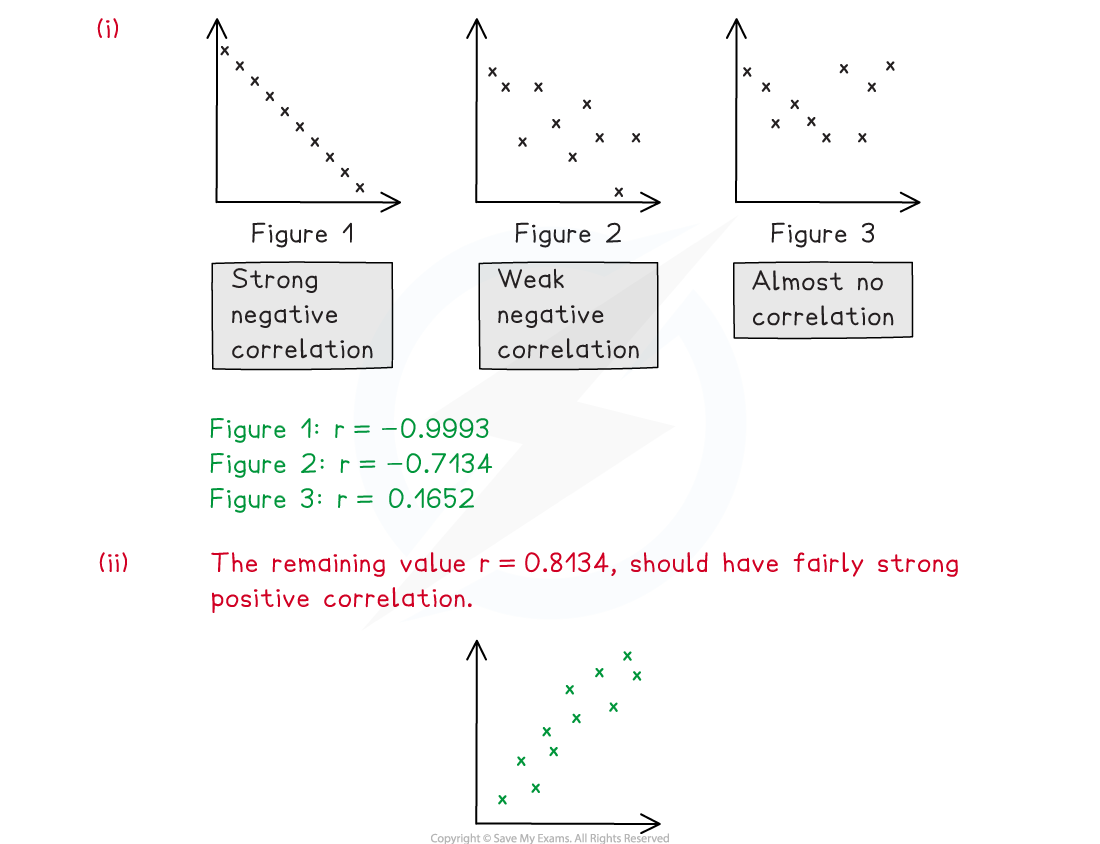### Non-linear Regression

At AS Level you learned how to use linear regression models to describe a relationship between two variables. However, it is possible for two variables to have a relationship that does not fit a linear model, but still shows a pattern based on exponential growth or decay. A linear regression model is only appropriate if the PMCC is close to 1 or -1.

#### What forms can non – linear regression models take?

• If a bivariate data set appears to have a non – linear relationship it could fit an exponential model

####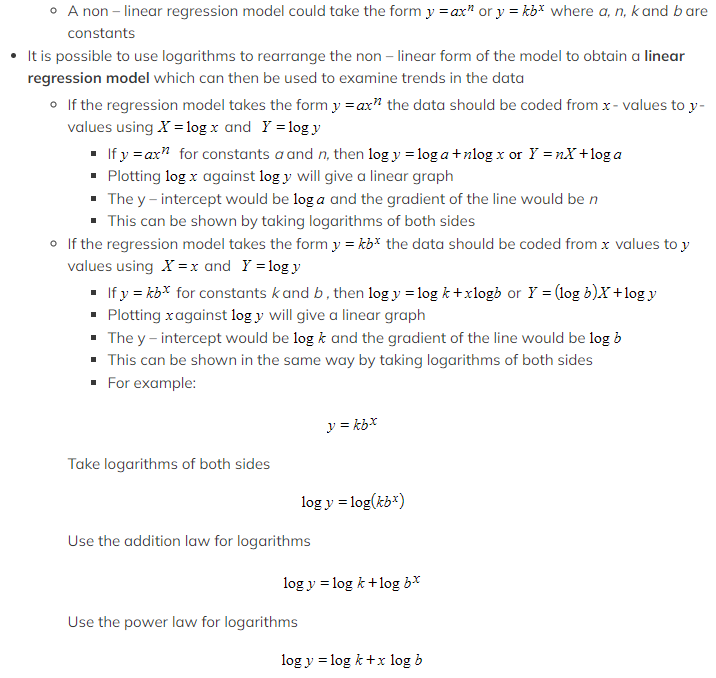How can non – linear regression models be used?

• Non – linear regression models can be used in much the same way as linear regression models
• By coding the original data using logarithms (changing the variables) a regression line of Y on X can be found
• This can be used to make predictions for data values that are within the range of the given data (interpolation)
• Making a prediction outside of the range of the given data is called extrapolation and should not be done

####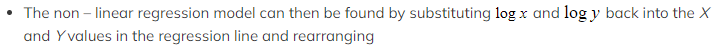Worked Example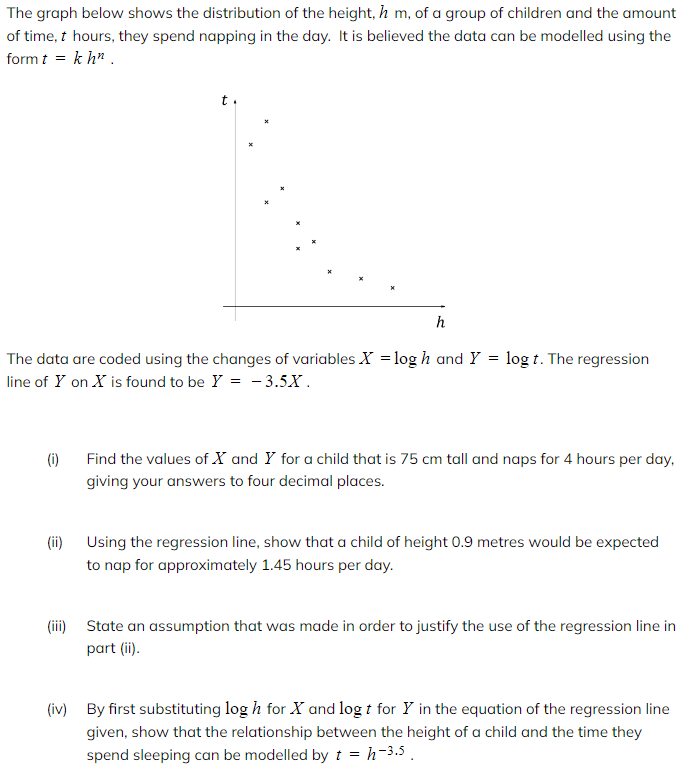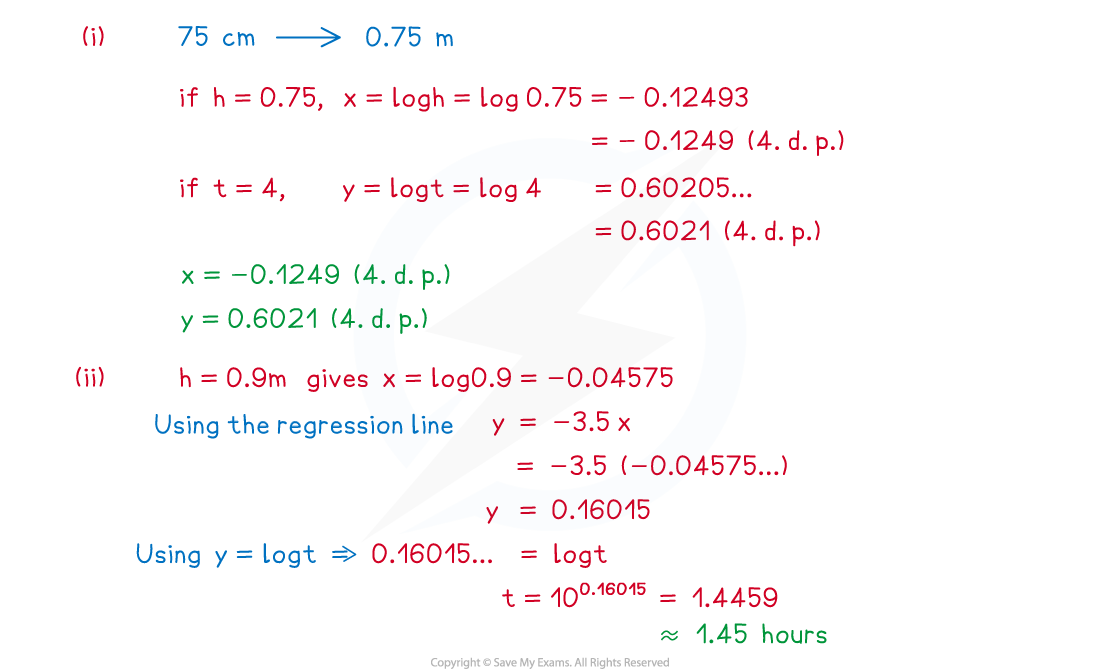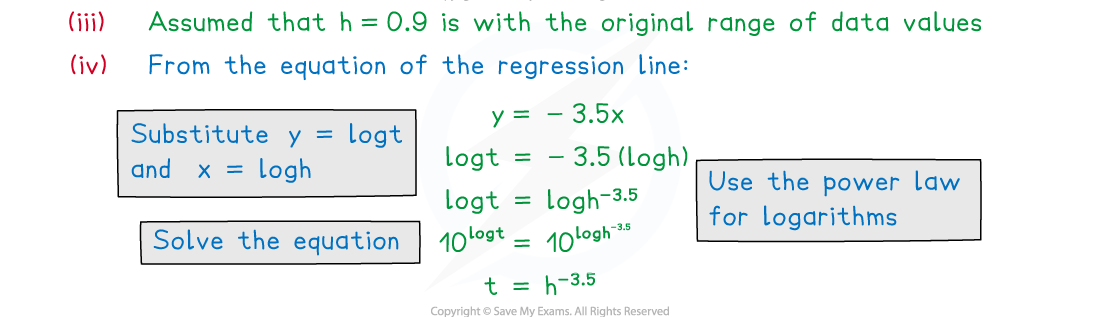#### Exam Tip